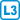## Monotone subsequences

Show that either a non-increasing subsequence of length $$a + 1$$ or a non-decreasing subsequence of length $$b + 1$$ can be found in each sufficiently sequence of integers.

• #### Solution

For a given sequence $$(a_1)_{i=1}^ n$$ we color the edge $$(i, j)$$, $$i < j$$ of the graph $$K_n$$ blue if $$a_i < a_j$$ and otherwise red.

If $$n > R (a + 1, b + 1)$$, then the elements of the sequence corresponding to the vertices of the monochromatic complete subgraph form the sought monotone subsequence.

• #### Variant

Show that such monotone subsequence exists in each sequence of length $$ab + 1$$.